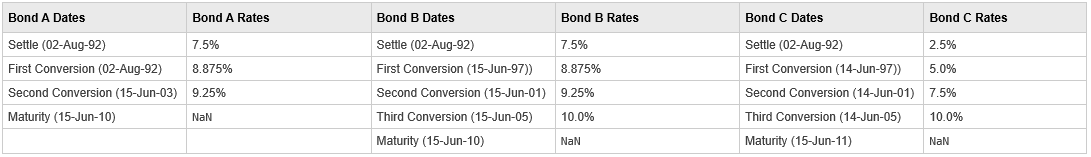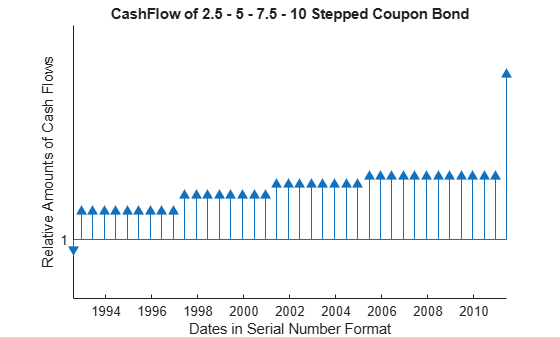# stepcpncfamounts

Cash flow amounts and times for bonds and stepped coupons

## Syntax

``````[CFlows,CDates,CTimes] = stepcpncfamounts(Settle,Maturity,ConvDates,CouponRates)``````
``[CFlows,CDates,CTimes] = stepcpncfamounts(___,Period,Basis,EndMonthRule,Face)``

## Description

example

``````[CFlows,CDates,CTimes] = stepcpncfamounts(Settle,Maturity,ConvDates,CouponRates)``` returns matrices of cash flow amounts, cash flow dates, and time factors for a portfolio of `NUMBONDS` stepped-coupon bonds.```

example

````[CFlows,CDates,CTimes] = stepcpncfamounts(___,Period,Basis,EndMonthRule,Face)` adds additional optional arguments.```

## Examples

collapse all

This example generates stepped cash flows for three different bonds, all paying interest semiannually. The life span of the bonds is about 18–19 years each:

• Bond A has two conversions, but the first one occurs on the settlement date and immediately expires.

• Bond B has three conversions, with conversion dates exactly on the coupon dates.

• Bond C has three conversions, with some conversion dates not on the coupon dates. It has the longest maturity. This case illustrates that only cash flows for full periods after conversion dates are affected, as illustrated here:The following table illustrates the interest-rate characteristics of this bond portfolio.Define the bond specifications.

```Settle = datenum('02-Aug-1992'); ConvDates = [datenum('02-Aug-1992'), datenum('15-Jun-2003'),... nan; datenum('15-Jun-1997'), datenum('15-Jun-2001'),... datenum('15-Jun-2005'); datenum('14-Jun-1997'), datenum('14-Jun-2001'),... datenum('14-Jun-2005')]; Maturity = [datenum('15-Jun-2010'); datenum('15-Jun-2010'); datenum('15-Jun-2011')]; CouponRates = [0.075 0.08875 0.0925 nan; 0.075 0.08875 0.0925 0.1; 0.025 0.05 0.0750 0.1]; Basis = 1; Period = 2; EndMonthRule = 1; Face = 100;```

Use `stepcpncfamounts` to compute cash flows and timings.

`[CFlows, CDates, CTimes] = stepcpncfamounts(Settle, Maturity, ConvDates, CouponRates)`
```CFlows = 3×39 -1.1639 4.4375 4.4375 4.4375 4.4375 4.4375 4.4375 4.4375 4.4375 4.4375 4.4375 4.4375 4.4375 4.4375 4.4375 4.4375 4.4375 4.4375 4.4375 4.4375 4.4375 4.4375 4.4375 4.6250 4.6250 4.6250 4.6250 4.6250 4.6250 4.6250 4.6250 4.6250 4.6250 4.6250 4.6250 4.6250 104.6250 NaN NaN -0.9836 3.7500 3.7500 3.7500 3.7500 3.7500 3.7500 3.7500 3.7500 3.7500 3.7500 4.4375 4.4375 4.4375 4.4375 4.4375 4.4375 4.4375 4.4375 4.6250 4.6250 4.6250 4.6250 4.6250 4.6250 4.6250 4.6250 5.0000 5.0000 5.0000 5.0000 5.0000 5.0000 5.0000 5.0000 5.0000 105.0000 NaN NaN -0.3279 1.2500 1.2500 1.2500 1.2500 1.2500 1.2500 1.2500 1.2500 1.2500 2.5000 2.5000 2.5000 2.5000 2.5000 2.5000 2.5000 2.5000 3.7500 3.7500 3.7500 3.7500 3.7500 3.7500 3.7500 3.7500 5.0000 5.0000 5.0000 5.0000 5.0000 5.0000 5.0000 5.0000 5.0000 5.0000 5.0000 5.0000 105.0000 ```
```CDates = 3×39 727778 727913 728095 728278 728460 728643 728825 729008 729191 729374 729556 729739 729921 730104 730286 730469 730652 730835 731017 731200 731382 731565 731747 731930 732113 732296 732478 732661 732843 733026 733208 733391 733574 733757 733939 734122 734304 NaN NaN 727778 727913 728095 728278 728460 728643 728825 729008 729191 729374 729556 729739 729921 730104 730286 730469 730652 730835 731017 731200 731382 731565 731747 731930 732113 732296 732478 732661 732843 733026 733208 733391 733574 733757 733939 734122 734304 NaN NaN 727778 727913 728095 728278 728460 728643 728825 729008 729191 729374 729556 729739 729921 730104 730286 730469 730652 730835 731017 731200 731382 731565 731747 731930 732113 732296 732478 732661 732843 733026 733208 733391 733574 733757 733939 734122 734304 734487 734669 ```
```CTimes = 3×39 0 0.7377 1.7377 2.7377 3.7377 4.7377 5.7377 6.7377 7.7377 8.7377 9.7377 10.7377 11.7377 12.7377 13.7377 14.7377 15.7377 16.7377 17.7377 18.7377 19.7377 20.7377 21.7377 22.7377 23.7377 24.7377 25.7377 26.7377 27.7377 28.7377 29.7377 30.7377 31.7377 32.7377 33.7377 34.7377 35.7377 NaN NaN 0 0.7377 1.7377 2.7377 3.7377 4.7377 5.7377 6.7377 7.7377 8.7377 9.7377 10.7377 11.7377 12.7377 13.7377 14.7377 15.7377 16.7377 17.7377 18.7377 19.7377 20.7377 21.7377 22.7377 23.7377 24.7377 25.7377 26.7377 27.7377 28.7377 29.7377 30.7377 31.7377 32.7377 33.7377 34.7377 35.7377 NaN NaN 0 0.7377 1.7377 2.7377 3.7377 4.7377 5.7377 6.7377 7.7377 8.7377 9.7377 10.7377 11.7377 12.7377 13.7377 14.7377 15.7377 16.7377 17.7377 18.7377 19.7377 20.7377 21.7377 22.7377 23.7377 24.7377 25.7377 26.7377 27.7377 28.7377 29.7377 30.7377 31.7377 32.7377 33.7377 34.7377 35.7377 36.7377 37.7377 ```

Visualize the third bond's cash flows (2.5 - 5 - 7.5 - 10) using the `cfplot` function.

```cfplot(CDates(3,:),CFlows(3,:)); xlabel('Dates in Serial Number Format') ylabel('Relative Amounts of Cash Flows') title('CashFlow of 2.5 - 5 - 7.5 - 10 Stepped Coupon Bond')```## Input Arguments

collapse all

Settlement date, specified either as a scalar or `NSTP`-by-`1` vector using serial date numbers or date character vectors.

`Settle` must be earlier than `Maturity`.

Data Types: `double` | `char`

Maturity date, specified as a scalar or an `NSTP`-by-`1` vector using serial date numbers or date character vectors that represent the maturity date for each bond.

Data Types: `double` | `char` | `string` | `datetime`

Conversion dates, specified as a `NSTP`-by-max(`NCONV`) matrix using serial date numbers or date character vectors containing conversion dates after `Settle`. The size of the matrix is equal to the number of instruments by the maximum number of conversions. Fill unspecified entries with `NaN`.

Data Types: `double` | `char`

Bond coupon rate, specified as an `NSTP`-by-max(`NCONV+1`) matrix containing coupon rates for each bond in the portfolio in decimal form. The matrix size is equal to the number of instruments by maximum number of conversions + 1. First column of this matrix contains rates applicable between `Settle` and the first conversion date (date in the first column of `ConvDates`). Fill unspecified entries with `NaN`

`ConvDates` has the same number of rows as `CouponRates` to reflect the same number of bonds. However, `ConvDates` has one less column than `CouponRates`. This situation is illustrated by

```Settle---------ConvDate1-----------ConvDate2------------Maturity Rate1               Rate2                 Rate3```

Data Types: `double`

(Optional) Coupons per year, specified as an `NSTP`-by-`1` vector. Values for `Period` are `1`, `2`, `3`, `4`, `6`, and `12`.

Data Types: `double`

(Optional) Day-count basis of each instrument, specified as an `NSTP`-by-`1` vector.

• 0 = actual/actual

• 1 = 30/360 (SIA)

• 2 = actual/360

• 3 = actual/365

• 4 = 30/360 (PSA)

• 5 = 30/360 (ISDA)

• 6 = 30/360 (European)

• 7 = actual/365 (Japanese)

• 8 = actual/actual (ICMA)

• 9 = actual/360 (ICMA)

• 10 = actual/365 (ICMA)

• 11 = 30/360E (ICMA)

• 12 = actual/365 (ISDA)

• 13 = BUS/252

Data Types: `double`

(Optional) End-of-month rule flag for generating dates when `Maturity` is an end-of-month date for a month having 30 or fewer days, specified for each bond as a nonnegative integer [`0`, `1`] using a `NSTP`-by-`1` vector.

• `0` = Ignore rule, meaning that a payment date is always the same numerical day of the month.

• `1` = Set rule on, meaning that a payment date is always the last actual day of the month.

Data Types: `logical`

(Optional) Face value, specified for each bond as an `NSTP`-by-`1` vector of nonnegative face values.

Data Types: `double`

## Output Arguments

collapse all

Cash flow amounts, returned as a vector where the first entry in each row vector is the (negative) accrued interest due at settlement. If no accrued interest is due, the first column is zero.

Cash flow dates, returned as vector in serial date number form. At least two columns are always present: one for settlement and one for maturity.

Time factor for the SIA semiannual price/yield conversion, returned as a vector. The SIA semi-annual price/yield conversion is ```DiscountFactor = (1 + Yield/2).^(-TFactor)```. Time factors are in units of semiannual coupon periods. For ISMA conventions: ```DiscountFactor = (1 + Yield).^(-TFactor)```. Time factors are in units of annual coupon periods. In computing time factors, use SIA actual/actual conventions for all time factor calculations.

Note

For bonds with fixed coupons, use `cfamounts`. If you use a fixed-coupon bond with `stepcpncfamounts`, MATLAB® software generates an error.

## Version History

Introduced before R2006a